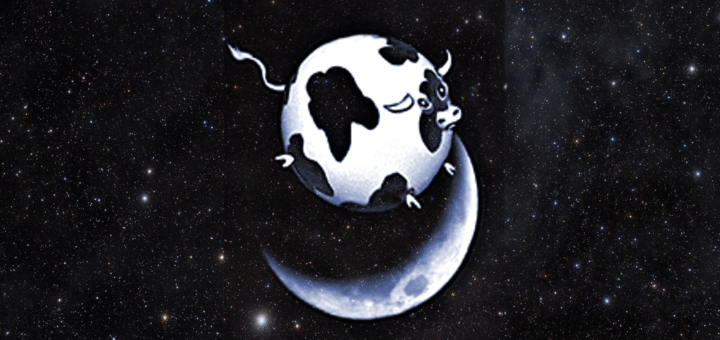Spherical Cow

In Science by Brian Koberlein1 Comment

There’s an old joke in physics where a farmer wants to increase milk production, and asks his physicist neighbor for advice. She agrees to think about the problem, and after a week comes back declaring she’s found a brilliant solution. “The first step is to get some spherical cows that can breed in a vacuum.” The point of the joke is physicists often make wildly unphysical assumptions when creating physical models, such as assuming cows are spherical. While that might seem like a poor way to do science, it is actually a powerful tool.

If you’ve ever taken a physics course, you’ve seen this method used. When calculating the motion of a baseball, for example, you start with some basic assumptions: the ground is perfectly flat (it isn’t); gravitational acceleration is the same regardless of height (not quite); the ball experiences no air resistance (really?). We know none of these assumptions are correct, so why make them?

One reason is that they make the problem solvable by beginning students. If you had to include variable gravity, planetary curvature and air resistance you would have a problem that can’t be solved by hand. All of these complications would make it difficult to demonstrate basic principles of gravity and motion. Another reason is that the effects we ignore don’t have a significant effect on the behavior of the ball.  If the ball doesn’t go too high, or too fast, then the answer found by the simple problem is pretty close to the actual answer.  It is good enough that you can use the simple answer in high stakes labs.

And therein lies its power.  By making simplifying assumptions we can test if our understanding of the underlying physics is correct.  Take the motion of the planets, for example. Most of the mass in the solar system is contained in the Sun.  Even Jupiter is tiny in comparison. So we can assume that the Sun and planets are all spherical, and that only the Sun exerts its gravity on the planets. When you make that assumption and calculate planetary motion, what you get is Kepler’s laws of planetary motion, which is a pretty good approximation.  If our understanding of gravity were wrong, then our simple model wouldn’t work.

We’ve done the same thing in other areas of astrophysics. The first models of stars assume they were simply large balls of gas in gravitational equilibrium. The first models of cosmology assumed that matter is evenly spread throughout the cosmos.  Neither of these assumptions were close to correct, but they were good starting points.  They demonstrated that we were on the right track before we delved into the complicated details.

There’s a famous quote attributed to Einstein that states scientists should make their theories “as simple as possible, but not simpler.” But there is power to be found in models that are “too” simple, because they pave the road toward deeper understanding.

1.